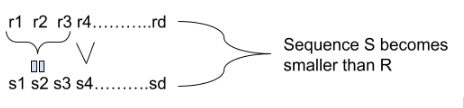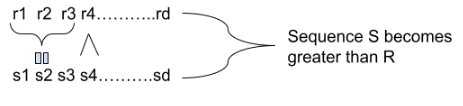# Introduction to Digit DP

## Introduction

Suppose you have two integers, 'X' and 'Y,' and you are required to count the number of integers' Z' which satisfy the given constraints, and Z must lie in the range X to Y, both inclusive.

If and are small(1 <= X, Y <= 10^6), you can iterate over all the numbers from to Y and check which satisfies the given constraints and keeps their count. But if X and Y are large (1 <= X, Y <= 10^18), then this approach is inefficient and gives the verdict "Time Limit Exceeded." So for such cases, we use Digit DP. By the Term "Digit DP," it's clear that we will apply Dynamic Programming on digits.

## Approach

Let Solve(L, R) give you the solution for the range L to R. For the time being, suppose L is zero. So, to solve for 0 to R, we have to count the total number of non-negative integers Z such that Z satisfies the given constraints and Z is less than or equal to R.

So, if we can find the solution for 0 to X - 1 and 0 to Y, then their difference will give the solution for range X to Y.

Solve(X, Y) = Solve(0, Y) - Solve(0, X - 1)

### How to solve for range zero to R??

Let d be the number of digits in the decimal representation of integer R (without leading zeros). Any number less than equal to R can't have the numbers of digits greater than d in its decimal representation. If there is a number less than R having less than d number of digits, we will add leading zeros to it, so we can say that every number less than R has exactly d number of digits in its decimal representation.

Let's consider each number as a sequence of digits. Let's assume that sequence r1,r2,r3,........, rd represents integer R.

Let 'S' be another sequence. S is initially empty.

We will recursively add digits to the next position of S, but we can't add any random digit from 0 to 9 to the sequence. We have to make sure that number is always less than or equal to R.

The index 0 is the most significant digit, and the index d - 1 is the least significant digit. So, if we want to place a digit at index 'i' and index 0 to i - 1 is already filled, then -

1. We can put any digit at position 'i' if there exists at least one index 'j' from 0 to 'i' such that S[j] < r[j] because the sequence is already smaller than R, so whatever we add won't create any difference.2. But if no such 'j' exists, we can't put a digit greater than r[i] because the number will become greater than R.So, we have to pass the next 'index to fill' and the 'current sequence' as a parameter to the recursive call.

This recursive solution is inefficient because we must pass the whole sequence whenever we make the recursive call. But there is no need to pass the entire sequence. We can use a boolean variable 'f' to check whether the current sequence is less than R or not. If f = 1, then the sequence is already smaller than R, and we can add any digit to the next index, but if f = 0, we can't.

So, we can build the sequence, but we have forgotten that the number formed by the sequence must satisfy the given constraints. For that, Let's take an example problem.

## Example Problem

Given two integers, X and Y., Your task is to find the count of integers Z such that digit t occurs exactly k times in Z.

Constraints : 1 <= X , Y <= 10^18,

0 <= t <= 9,

1 <= K <= 18

## Solution

As discussed earlier, we have to find the solution for the range 0 to L - 1 and 0 to R to solve this problem.

Now, to keep the count of digit 't' in the sequence, we need another parameter, 'num.' Whenever we place digit t at any index in our sequence, we increment the num in the next recursive call.

### States of DP

So, the final states of DP are current position (index at which we place the next digit), whether the number is already smaller than R or not (boolean variable f), and frequency of digit t till now (num).

### Base Case

If the current index is equal to d, we have built the whole sequence, and then we need to check whether the num is equal to k or not. If num is equal to k, we return 1. Otherwise, we return 0

### Code

``````#include <bits/stdc++.h>
#define int long long
using namespace std;
int X, Y, t, k;
vector <int> r; //sequence r - represents R
int dp; //3D array to memorize the states

/*
index - current index
num - frequency of digit t
f - boolean variable to check the current sequence is less than R or not
*/

int rec(int index, int num, int f){

if(index == r.size()){   //Base case
if(num == k)
return 1;
else
return 0;
}
if(dp[index][num][f] != -1)   //to avoid overlapping
return dp[index][num][f];
int res = 0;
if(f == 0){
/*
digits we placed so far matches with the prefix of r
so, we can't place digit > r[index] at the current index.
*/
for(int i = 0; i <= r[index]; ++i){
if(i == r[index]){
/*
i == r[index] and f == 0, so the digits we placed
still match with prefix of r, so f = 0
*/
res += rec(index + 1, num + (i == t), 0);

}
else{
/*
i < r[index] so, f = 1
*/
res += rec(index + 1, num + (i == t), 1);
}
}
}
else{
/*
f = 1, so the sequence is already smaller than r
so we can place any digit at the current index.
*/
for(int i = 0; i <= 9; ++i){
res += rec(index + 1, num + (i == t), 1);
}
}
return dp[index][num][f] = res;

}
int Solve(int R){
r.clear();
while(R > 0){
r.push_back(R%10);
R /= 10;
}
//to store the digits of R in the decreasing order of  significance we have to reverse the sequence r
reverse(r.begin(), r.end());
memset(dp, -1, sizeof(dp));
return rec(0, 0, 0);
}
signed main(){
cin >> X >> Y >> t >> k;   //range - [X, Y], digit - t, frequency - k
cout << Solve(Y) - Solve(X - 1);  //Solve(X, Y) = Solve(0, Y) - Solve(0, X - 1)
return 0;
}``````

### Time Complexity

The overall complexity is O(index * frequency * f * 10). The factor of ten is included because, at each state, we will try to place all the ten digits from 0 to 9 at the current index.

XY can't be greater than 10^18, so the maximum number of digits in X and Y can be 19, K can't be greater than 20, and the boolean variable f can be 0 or 1. so the total number of iteration can be 10 * 20 * 19 * 2 ~ 10^5.

### Space complexity

We have to use a 3D array to memorize each state. Each Dimension represents one parameter, so the total space complexity is O(index * f * frequency).

## FAQs

1. What are states in dynamic programming?
The states of DP are the subproblems to the more significant problem. Each state is a subset of the input data used to build the result for the whole problem input.

2. What is the base case?
The base case is the precalculated value for the smaller cases. It is used to terminate the recursive calls.

## Key Takeaways

This article covered the basics of digit DP and an example problem. Having a good hold on Dynamic Programming is very important for cracking coding interviews at MAANG.

Check out the coding ninjas master class on dynamic programming for getting a better hold on dynamic programming.

To learn more about such data structures and algorithms, Codestudio is a one-stop destination. This platform will help you to improve your coding techniques and give you an overview of interview experiences in various product-based companies by providing Online Mock Test Series and many more benefits.

Happy learning!